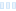General Discussions
Using Tag to Create a Control Chart

I'd like to use a tag to create an Upper Control Limit (UCL) and a Lower Control Limit (LCL). This involves calculating the mean and standard deviation for a period of time. The formulas would be:

UCL = mean + (3 x (Standard Deviation))
LCL = mean - (3 x (Standard Deviation))What would be the best approach to do this? I was looking at doing an I/O Calc tag, but the avg() and SDev() functions are not availalbe. I also tried looking at the Historical Statistics tag but all I can do is calculate the average over a period of time, but not the standard deviation. Any insights would be helpful, thanks!

I&#039;d like to use a tag to create an Upper Control Limit (UCL) and a Lower Control Limit (LCL). This involves calculating the mean and standard deviation for a period of time. The formulas would be: UCL = mean + (3 x (Standard Deviation)) LCL = mean - (3 x (Standard Deviation))![65047a8a0dacd](serve/attachment&amp;path=65047a8a0dacd) What would be the best approach to do this? I was looking at doing an I/O Calc tag, but the avg() and SDev() functions are not availalbe. I also tried looking at the Historical Statistics tag but all I can do is calculate the average over a period of time, but not the standard deviation. Any insights would be helpful, thanks!

The VTScada script language includes SDev() and Mean() functions, but they operate on elements in an array which is typically unavailable in a I/O and Calc tag. However, you can write Script tag to collect the raw data into an array and then calculate the SDev value. You'll probably want to write a separate Script tag for the Mean value. There's an example Script tag in the Help files to get you started.

The VTScada script language includes SDev() and Mean() functions, but they operate on elements in an array which is typically unavailable in a I/O and Calc tag. However, you can write Script tag to collect the raw data into an array and then calculate the SDev value. You&#039;ll probably want to write a separate Script tag for the Mean value. There&#039;s an example Script tag in the Help files to get you started.
32
1
2
live preview
enter atleast 10 characters
WARNING: You mentioned %MENTIONS%, but they cannot see this message and will not be notifiedSaving...
Saved
With selected deselect posts show selected posts
All posts under this topic will be deleted ?
Pending draft ... Click to resume editing